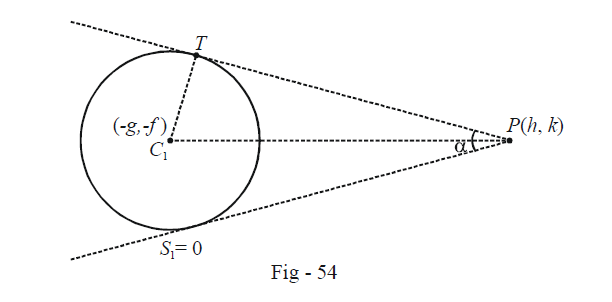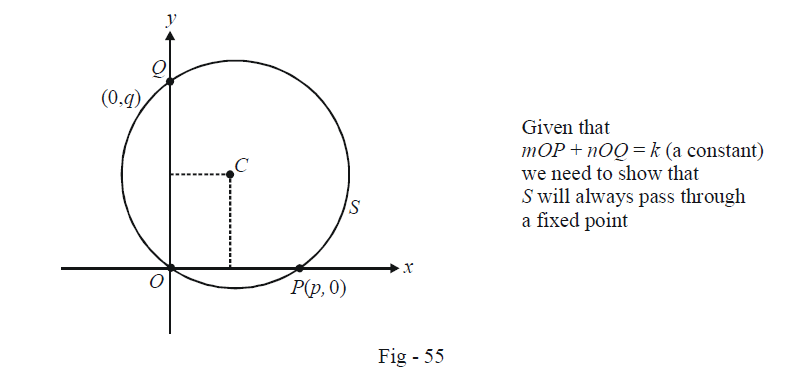# Circles Set-3

Go back to  'SOLVED EXAMPLES'

Example –7

Let $${S_1} = 0$$ and $${S_2} = 0$$ be two circles with radii $${r_1}$$  and $${r_2}$$ respectively. Find the locus of the point P at which the two circles subtend equal angles.

Solution: Consider  $${S_1} = 0$$ which subtends an angle $$\alpha$$ (say) at $$P(h,k)$$We have,

$${C_1}T = {r_1}$$ and $$PT = \sqrt {{S_1}(h,k)}$$

Thus, in $$\Delta {C_1}PT,$$ we have

$\tan \frac{\alpha }{2} = \frac{{{r_1}}}{{\sqrt {{S_1}(h,k)} }}\,\,\,\,\,\,\,\,\,\,\,\,\,\,\,\,\,\,\,\,\,\,\,\,\,\,\,\,\,\,\,\,\,\,\,\,\,\,\,\,\,\,\,\,\,\,\,\,\,\,\,\,\,\,...(1)$

We can write an exactly analogous equation for $${S_2}$$ which subtends the same angle $$\alpha$$ at P:

$\tan \frac{\alpha }{2} = \frac{{{r_2}}}{{\sqrt {{S_2}(h,k)} }}\,\,\,\,\,\,\,\,\,\,\,\,\,\,\,\,\,\,\,\,\,\,\,\,\,\,\,\,\,\,\,\,\,\,\,\,\,\,\,\,\,\,\,\,\,\,\,\,\,\,\,\,\,\,...\left( 2 \right)$

From (1) and (2), we have

$\frac{{{r_1}}}{{\sqrt {{S_1}(h,k)} }} = \frac{{{r_2}}}{{\sqrt {{S_2}(h,k)} }}$

Using $$(x,y)$$  instead of $$(h,k),$$ we obtain the required locus as

$\frac{{{S_1}(x,y)}}{{r_1^2}} = \frac{{{S_2}(x,y)}}{{r_2^2}}$

Example –8

Circles are drawn passing through the origin O to intersect the co-ordinate axes at points P and Q such that $$m \cdot OP + n \cdot OQ$$ is a constant. Show that the circles pass through a fixed point other than the origin.

Solution: Consider one such circle as shown in the figure below:We have $$OP = p$$ and $$OQ = q.$$ Thus,

$mp + nq = k\,\,\,\,\,\,\,\,\,\,\,\,\,\,\,\,\,\,\,\,\,\,\,\,\,\,\,\,\,\,\,\,\,\,\,\,\,\,\,\,...(1)$

Now, observe that the centre C of the circle will be \begin{align} \left( {\frac{p}{2},\frac{q}{2}} \right)\end{align}  and its radius will be OC. Thus, the equation of the circle is

\begin{align}&\,\,\,\,\,\,\,\,\,\,\,\,\,\,{\left( {x - \frac{p}{2}} \right)^2} + {\left( {y - \frac{q}{2}} \right)^2} = \frac{{{p^2}}}{4} + \frac{{{q^2}}}{4}\\&\Rightarrow \quad {x^2} + {y^2} - px - qy = 0\,\,\,\,\,\,\,\,\,\,\,\,\,\,\,\,\,\,\,\,\,\,\,...\left( 2 \right)\end{align}

From (1), we can find q in terms of p and substitute in (2) so that (2) becomes

\begin{align}&\,\,\,\,\,\,\,\,\,\,\,\,\,\,\,\,\,{x^2} + {y^2} - px + \left( {\frac{{mp - k}}{n}} \right)y = 0\\&\Rightarrow \quad \{ n({x^2} + {y^2}) - ky\} + p\{ - nx + my\} = 0\end{align}

We see that the variable circle can be written as

${S_0} + p{L_0} = 0,p \in \mathbb{R}$

where $${S_0} \equiv n({x^2} + {y^2}) - ky = 0$$ and $${L_0} \equiv - nx + my = 0$$. Thus, the variable circle will always pass through the two intersection points of $${S_0}$$ and $${L_0}$$, one of them obviously being the origin. The other fixed point can be obtained by simultaneously solving the equations of $${S_0}$$ and $${L_0}$$ to be

$\left( {\frac{{mk}}{{{m^2} + {n^2}}},\frac{{nk}}{{{m^2} + {n^2}}}} \right)$

Example –9

The two curves

$\begin{array}{l}{S_1}:a{x^2} + 2hxy + b{y^2} - 2gx - 2fy + c = 0\\{S_2}:a'{x^2} - 2hxy + (a' + a - b){y^2} - 2g'x - 2f'y + c = 0\end{array}$

intersect at four concyclic points and Let P be the point \begin{align}\left( {\frac{{g' + g}}{{a' + a}},\frac{{f' + f}}{{a' + a}}} \right).\end{align} Find the value of \begin{align}\frac{{P{A^2} + P{B^2} + P{C^2}}}{{P{D^2}}}.\end{align}

Solution: From what we’ve learnt in this chapter, we can write the equation of an arbitrary curve S passing through the intersection points of two given curves, here $${S_1}$$ and $${S_2}$$, as

$S \equiv {S_1} + \lambda {S_2} = 0) \; where\; \lambda \in \mathbb{R}$

Here,

$S:(a + \lambda a'){x^2} + 2h(1 - \lambda )xy + (b + \lambda (a' + a - b)){y^2} - 2x(g + g'\lambda ) - 2y(f + f') + c(1 + \lambda ) = 0\,...\left( 1 \right)$ Since this curve S passes through the four concyclic points $$A,\,\,B,\,\,C,\,\,D$$ its equation in (1) must be that  of a circle. Thus, we impose the necessary constraints:

\begin{align}&{\text{Coeff}}{\text{. of }}\;{x^2} = {\text{Coeff}}{\text{. of }}\;{y^2} \quad \Rightarrow \quad a + \lambda a' = b + \lambda (a' + a -b)\\&\qquad\qquad\qquad\qquad\qquad\qquad\;\;\Rightarrow \quad \lambda = 1\\&{\text{Coeff}}{\text{. of }}xy = 0 \qquad \qquad\quad\;\; \Rightarrow \quad \lambda = 1\end{align}

Thus, for $$\lambda = 1,$$ the equation in (1) represents a circle. This equation now becomes :

$S:(a + a'){x^2} + (a + a'){y^2} - 2(g + g')x - 2(f + f')y + 2c = 0$

The centre of S therefore is \begin{align}\left( {\frac{{g + g'}}{{a + a'}},\frac{{f + f'}}{{a + a'}}} \right).\end{align}  But this is the same as the point P!. Thus, P is the centre of the circle passing through $$A,\,\,B,\,\,C,\,\,D$$  so that

$PA = PB = PC = PD$

Thus, we simply have

\begin{align}\frac{{P{A^2} + P{B^2} + P{C^2}}}{{P{D^2}}} = 3\end{align}

Learn from the best math teachers and top your exams

• Live one on one classroom and doubt clearing
• Practice worksheets in and after class for conceptual clarity
• Personalized curriculum to keep up with school